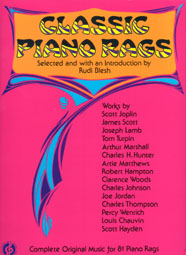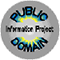PD InfoPublic Domain Information Project

Public Domain Sheet Music Books# Classic Piano Rags

Out of Print

• Used Copies Usually in Stock - Call for Price 770-993-8074
• Also check Amazon.com - eBay - Used Book Sellers
• Selected and with an Introduction by Rudi Blesh
• xi + 364 pages9" x 12"Paperback
• Dover Publications, Inc.
• ISBN: 0486204693

This book contains Sheet Music reprints of first or early editions of 81 piano rags from 1897 to 1922.   Included are works written by ragtime greats such as Scott Joplin, James Scott, and Joseph Lamb.  All 81 selections are piano rags and therefore have no lyrics.  The publisher thanks Mr. Trebor Jay Tichenor and Mr. William Lloyd Keepers for the generous loan of sheet music to make these reprints possible.

PUBLIC DOMAIN STATUS: All selections are reprints of original or early editions with copyright date and publisher shown.  All are believed to be in the public domain in the United States.   Public domain status may not apply in countries outside of the United States where copyright protection may exist.

SONGS INCLUDED:  =][=  Agitation Rag (1915)  =][=  American Beauty Rag (1913)  =][=  Ashy Africa (1903)  =][=  Back to Life (1905)  =][=  A Black Smoke (1902)  =][=  Blue Goose Rag (1916)  =][=  Bohemia Rag (1919)  =][=  Bowery Buck (1899)  =][=  A Breeze from Alabama (1902)  =][=  Broadway Rag (1922)  =][=  The Buffalo Rag (1904)  =][=  The Cascades (1904)  =][=  Cataract Rag (1914)  =][=  Champagne Rag (910)  =][=  The Chrysanthemum (1904)  =][=  Cleopha (1902)  =][=  Cotton Bolls (1901)  =][=  Cum-Bac Rag (1911)  =][=  Don't Jazz Me Rag (1921)  =][=  The Easy Winners (1901)  =][=  Efficiency Rag (1917)  =][=  Elite Syncopations (1902)  =][=  The Entertainer (1902)  =][=  Ethiopia Rag (1909)  =][=  Eugenia (1905)  =][=  Evergreen Rag (1915)  =][=  Excelsior Rag (1909)  =][=  The Favorite (1904)  =][=  Frog Legs Rag (1906)  =][=  Great Scott Rag (1909)  =][=  Ham And! (1908)  =][=  Harlem Rag (1897)  =][=  Heliotroupe Bouquet (1907)  =][=  Hilarity Rag (1910)  =][=  Kansas City Rag (1907)  =][=  Kinklets (1906)  =][=  The Lily Rag (1914)  =][=  Maple Leaf Rag (1899)  =][=  Modesty Rag (1920)  =][=  Nappy Lee (1903)  =][=  New Era Rag (1919)  =][=  Original Rags (1899)  =][=  Palm Leaf Rag (1903)  =][=  Paramount Rag (1917)  =][=  Pastime Rag No. 1 (1913)  =][=  Pastime Rag No. 2 (1913)  =][=  Pastime Rag No. 3 (1916)  =][=  Pastime Rag No. 4 (1920)  =][=  Pastime Rag No. 5 (1918)  =][=  Patricia Rag (1916)  =][=  Peace and Plenty Rag (1919)  =][=  The Peach (1908)  =][=  Peacherine Rag (1901)  =][=  Pegasus (1920)  =][=  The Pippin (1908)  =][=  Pleasant Moments (1909)  =][=  Possum and Taters-A Ragtime Feast (1900)  =][=  Queen of Love (1901)  =][=  Rag Sentimental (1918)  =][=  Rag-Time Nightmare, A (1900)  =][=  The Ragtime Betty (1909)  =][=  The Ragtime Dance (1906)  =][=  Ragtime Nightingale (1915)  =][=  Ragtime Oriole (1911)  =][=  Reflection Rag (1917)  =][=  Reindeer (1915)  =][=  Sensation (1908)  =][=  Sleepy Hollow Rag (1918)  =][=  Slippery Elm Rag (1912)  =][=  The Smiler (1907)  =][=  Something Doing (1903)  =][=  The St. Louis Rag (1903)  =][=  The Strenuous Life (1902)  =][=  Sunburst Rag (1909)  =][=  Sunflower Slow Drag (1901)  =][=  Swipesy Cake Walk (1900)  =][=  A Tennessee Tantalizer (1900)  =][=  Tickled to Death (1899)  =][=  Troubadour Rag (1919)  =][=  Victory Rag (1921)  =][=  Weeping Willow (1903)  =][=

### What We Do . . .770-993-8074